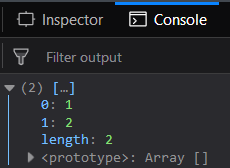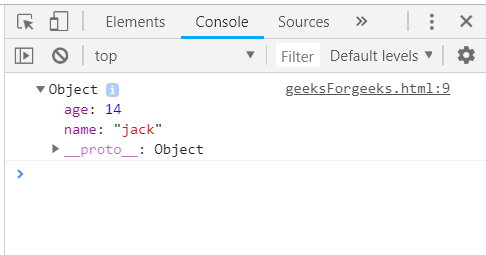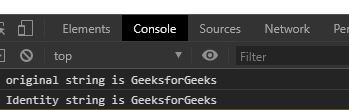# Features of Underscore.js

• Last Updated : 04 Jan, 2022

Underscore.js is a lightweight JavaScript library and not a complete framework that was written by Jeremy Ashkenas that provides utility functions for a variety of use cases in our day-to-day common programming tasks. It provides a lot of features that make our task easy to work with objects. It can be used directly inside a browser and also with Node.js.

Following are the main features of Underscore.js that make it so popular:

1. Arrays: It contains different functions for arrays to perform various operations on arrays such as to return first element, last element, intersection, difference, creating a copy of the array, etc.

Example: In this example, we will find the intersection of the passed arrays using Underscore.js _.intersection() Function.

## HTML

 ```<``html``>` `<``head``>``    ``<``script` `src``=``"https://cdnjs.cloudflare.com/ajax/libs/underscore.js/1.9.1/underscore-min.js"``>``    ````` `<``body``>``    ``<``script` `type``=``"text/javascript"``>``        ``console.log(_.intersection(``            ``[1, 2, 3, 4, 5],``            ``[1, 2, 3, 4, 6, 7],``            ``[1, 2, 6, 8, 9])``        ``);``    ````` ``

Output:2. Collections: Underscore.js Collection functions are used on arrays, objects, and array-like objects such as arguments, NodeList, and similar other types of elements.

Example: In this example, we will look at each element of the list and returns the first occurrence of the elements that satisfy the condition using Underscore.js _.find() Function.

## HTML

 ```<``html``>` `<``head``>``    ``<``script` `type``=``"text/javascript"` `src``=``"https://cdnjs.cloudflare.com/ajax/libs/underscore.js/1.9.1/underscore-min.js"``>``    ````` `<``body``>``    ``<``script` `type``=``"text/javascript"``>``        ``var oddNo = _.find([5, 6, 7, 8, 9, 10],``            ``function (num) {``                ``return num % 2 != 0;``            ``});``        ``console.log(oddNo);``    ````` ``

Output:

`5`

3. Functions: Underscore.js provides a number of functions that can be applied to the elements. These include the ability to bind a function to an object, wrap a function inside another function, memorize a given function by caching the result computed by the function, etc.

Example: In this example, we will return the first element of the array, i.e. the number at the zeroth index using Underscore.js _.first() Function.

## HTML

 ```<``html``>` `<``head``>``    ``<``script` `type``=``"text/javascript"` `src``=``"https://cdnjs.cloudflare.com/ajax/libs/underscore.js/1.9.1/underscore.js"``>``    ````` `<``body``>``    ``<``script` `type``=``"text/javascript"``>``        ``console.log(_.first([``            ``{ name: 'jack', age: 14 },``            ``{ name: 'jill', age: 15 },``            ``{ name: 'humpty', age: 16 }``        ``]));``    ````` ``

Output:4. Objects: Underscore.js provides a number of common objects related methods such as the key and values functions. These functions are used to return the list of all keys and values of the given object respectively.

Example: In this example, we will create a new object with the stated prototype and props using Underscore.js _.create() Function.

## HTML

 ```<``html``>` `<``head``>``    ``<``script` `src``=``"https://cdnjs.cloudflare.com/ajax/libs/underscore.js/1.9.1/underscore-min.js"``>``    ````` `<``body``>``    ``<``script``>``        ``var author_article = [``            ``{ author: 'Nidhi1352', articles: 792 },``            ``{ author: 'Nisha95', articles: 590 },``            ``{ author: 'Rohit01', articles: 450 }``        ``];` `        ``// Calling create method with its parameter``        ``var obj = _.create(author_article.prototype,``                    ``{ author: "Rahul096" });``        ``console.log(obj);``    ````` ``

Output:

`{"author":"Rahul096"}`

5. Utilities: Underscore.js provides various utility methods like Brandon, constant, times, mixin, etc.

Example: In this example, we will return the exact same replica of the value given to it as the argument using Underscore.js _.identity() Function.

## HTML

 ```<``html``>` `<``head``>``    ``<``script` `src``=``"https://cdnjs.cloudflare.com/ajax/libs/underscore.js/1.9.1/underscore-min.js"``>``    ````` `<``body``>``    ``<``script``>` `        ``// Creating a string``        ``let str = new String("GeeksforGeeks")` `        ``// _.identity function of underscore.js``        ``let copystr = _.identity(str)``        ``console.log(`original string is \${str}`)``        ``console.log(`Identity string is \${copystr}`)``    ````` ``

Output:My Personal Notes arrow_drop_up